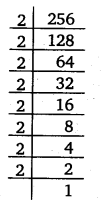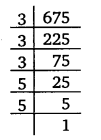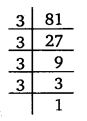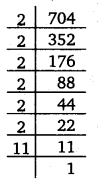# Class 8 Maths NCERT Solutions for Chapter – 7 Cubes and Cube Roots Ex – 7.1

## Cubes and Cube Roots

Question 1.
Which of the following numbers are not perfect cubes?
(i)
216
(ii) 128
(iii) 1000
(iv) 100
(v) 46656

Solution:
(i) Resolving 216 into prime factors, we find that 2
16 = 2x2x2 x 3x3x3
Clearly, the prime factors of 216 can be grouped into triples of equal factors and no factor is left over.
∴ 216 is a perfect cube.(ii) Resolving 128 into prime factors, we find that
128 = 2x2x2 x 2x2x2 x 2
Now, if we try to group together triples of equal factors, we are left with a single factor, 2.
∴ 128 is not a perfect cube.(iii) Resolving 1000 into prime factors, we find that
1000 = 10 x 10 x 10 = 2x5x2x5x2x5 = 2x2x2x 5x5x5
Clearly, the prime factors of 1000 can be grouped into triples of equal factors and no factor is left over.
∴ 1000 is a perfect cube.

(iv) Resolving 100 into prime factors, we find that
100 = 10×10=2x5x2x5=2x2x5x5
Clearly, the prime factors of 100 cannot be grouped into triples of equal
factors.
∴ 100 is not a perfect cube.

(v) Resolving 46656 into prime factors, we find that
46656 = 2 x 2 x 2 x 2 x 2 x 2 x 3 x 3 x3 x 3x3x3
Clearly, the prime factors of 46656 can be grouped into prime factors and no factor is left over.
∴ 46656 is a perfect cube.Question 2.
Find the smallest number by which each of the following numbers must be multiplied to obtain a perfect cube.
(i)
243
(ii) 256
(iii) 72
(iv) 675
(v) 100

Solution:
(i) Writing 243 as a product of prime factors, we have
243 = 3 x 3 x 3 x 3 x 3
Clearly, to make it a perfect cube, it must be multiplied by 3.(ii) Writing 256 as a product of prime factors, we have
256 = 2 x 2 x 2 x 2 x 2 x 2 x 2 x 2
Clearly, to make it a perfect cube, it must be multiplied by 2.(iii) Writing 72 as a product of prime factors, we have
72 = 2x2x2 x 3 x 3
Clearly, to make it a perfect cube, it must be multiplied by 3.(iv) Writing 675 as a product of prime factors, we have
675 = 3 x 3 x 3 x 5 x 5
Clearly, to make it a perfect cube, it must be multiplied by 5.(v) Writing 100 as a product of prime factors, we have
100 = 2 x 2 x 5 x 5
Clearly, to make it a perfect cube, it must be multiplied 2 x 5, i.e., 10.Question 3.
Find the smallest number by which each of the following numbers must he divided to obtain a perfect cube.
(i)
81
(ii) 128
(iii) 135
(iv) 192
(v) 704

Solution:
(i) Writing 81 as a product of prime factors, we have
81 = 3 x 3 x 3 x 3
Clearly, to make it a perfect cube, it must be divided by 3.(ii) Writing 128 as a product of prime factors, we have
128 = 2 x 2 x 2 x 2 x 2 x 2 x 2
Clearly, to make it a perfect cube, it must be divided by 2.(iii) Writing 135 as a product of prime factors, we have
135 = 3 x 3 x 3 x 5
Clearly, to make it a perfect cube, it must be divided by 5.(iv) Writing 192 as a product of prime factors, we have
192 = 2 x 2 x 2 x 2 x 2 x 2 x 3
Clearly, to make it a perfect cube, it must be divided by 3.(v) Writing 704 as a product of prime factors, we have
704 = 2 x 2 x 2 x 2 x 2 x 2 x 11
Clearly, to make it a perfect cube, it must be divided by 11.Question 4.
Parikshit makes a cuboid of plasticine of sides 5 cm, 2 cm, 5 cm. How many such cuboids will he need to form a cube?

Solution:
Volume of the cuboid = 5 x 2 x 5 = 2 x 5 x 5
To make it a cube, we require 2 x 2 x 5, i.e., 20 such cuboids.

#### Class 8 Maths NCERT Solutions for Chapter – 7 Cubes and Cube Roots Ex – 7.2

Lorem ipsum dolor sit amet, consectetur adipiscing elit. Phasellus cursus rutrum est nec suscipit. Ut et ultrices nisi. Vivamus id nisl ligula. Nulla sed iaculis ipsum.

Company Name